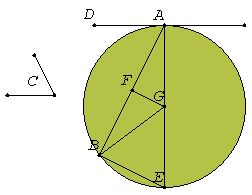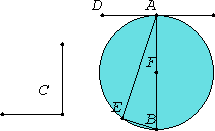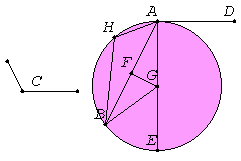# Proposition 33

On a given straight line to describe a segment of a circle admitting an angle equal to a given rectilinear angle.

Let AB be the given straight line, and the angle at C the given rectilinear angle.

It is required to describe on the given straight line AB a segment of a circle admitting an angle equal to the angle at C.

The angle at C is then acute, or right, or obtuse.I.23

First let it be acute as in the first figure. Construct the angle BAD equal to the angle at C on the straight line AB and at the point A. Therefore the angle BAD is also acute.

Draw AE at right angles to DA. Bisect AB at F. Draw FG from the point F at right angles to AB, and join GB.

I.4

Then, since AF equals FB, and FG is common, the two sides AF and FG equal the two sides BF and FG, and the angle AFG equals the angle BFG, therefore the base AG equals the base BG.

Therefore the circle described with center G and radius GA passes through B also.

Draw it as ABE, and join EB.

III.16,Cor.

Now, since AD is drawn from A, the end of the diameter AE, at right angles to AE, therefore AD touches the circle ABE.

III.32

Since then a straight line AD touches the circle ABE, and from the point of contact at A a straight line AB has been drawn across in the circle ABE, the angle DAB equals the angle AEB in the alternate segment of the circle.

But the angle DAB equals the angle at C, therefore the angle at C also equals the angle AEB.

Therefore on the given straight line AB the segment AEB of a circle has been described admitting the angle AEB equal to the given angle, the angle at C.Next let the angle at C be right, and let it be again required to describe on AB a segment of a circle admitting an angle equal to the right angle at C.

Let the angle BAD be constructed equal to the right angle at C, as is the case in the second figure. Bisect AB at F. Describe the circle AEB with center F and radius either FA or FB.

III.16 Cor.

Therefore the straight line AD touches the circle ABE, because the angle at A is right.

III.31

And the angle BAD equals the angle in the segment AEB, for the latter too is itself a right angle, being an angle in a semicircle.

But the angle BAD also equals the angle at C, therefore the angle AEB also equals the angle at C.

Therefore again the segment AEB of a circle has been described on AB admitting an angle equal to the angle at C.

Next, let the angle at C be obtuse.Construct the angle BAD equal to C on the straight line AB and at the point A as is the case in the third figure. Draw AE at right angles to AD. Bisect AB again at F. Draw FG at right angles to AB, and join GB.

I.4

Then, since AF again equals FB, and FG is common, the two sides AF and FG equal the two sides BF and FG, and the angle AFG equals the angle BFG, therefore the base AG equals the base BG.

Therefore the circle described with center G and radius GA also passes through B. Let it so pass, as AEB.

III.16 Cor.

Now, since AD is drawn at right angles to the diameter AE from its end, AD touches the circle AEB.

III.32

And AB has been drawn across from the point of contact at A, therefore the angle BAD equals the angle constructed in the alternate segment AHB of the circle.

But the angle BAD equals the angle at C.

Therefore the angle in the segment AHB also equals the angle at C.

Therefore on the given straight line AB the segment AHB of a circle has been described admitting an angle equal to the angle at C.

Q.E.F.

## Guide

This proposition is not used in the rest of the Elements.